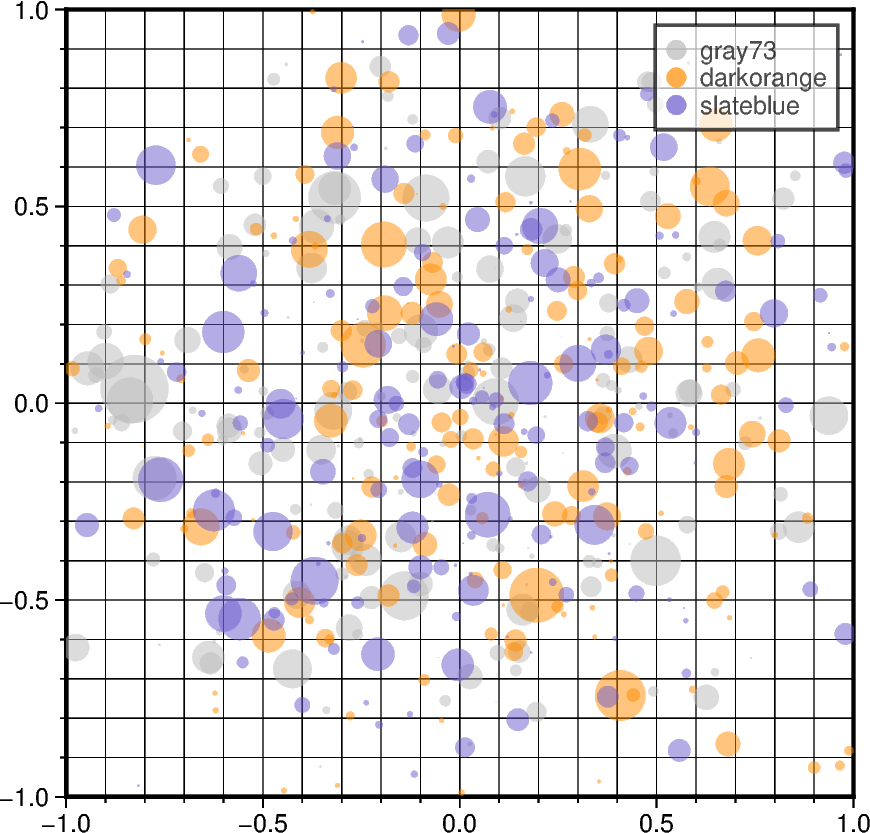# Scatter plots with a legend

To create a scatter plot with a legend one may use a loop and create one scatter plot per item to appear in the legend and set the label accordingly.

Modified from the matplotlib example: https://matplotlib.org/stable/gallery/lines_bars_and_markers/scatter_with_legend.html```import numpy as np
import pygmt

rng = np.random.default_rng(seed=19680801)
n = 200  # number of random data points

fig = pygmt.Figure()
fig.basemap(
region=[-1, 1, -1, 1],
projection="X10c/10c",
frame=["xa0.5fg", "ya0.5fg", "WSrt"],
)
for fill in ["gray73", "darkorange", "slateblue"]:
# Generate standard normal distributions centered on 0
# with standard deviations of 1
x = rng.normal(loc=0, scale=0.5, size=n)  # random x data
y = rng.normal(loc=0, scale=0.5, size=n)  # random y data
size = rng.normal(loc=0, scale=0.5, size=n) * 0.5  # random size, in cm

# plot data points as circles (style="c"), with different sizes
fig.plot(
x=x,
y=y,
style="c",
size=size,
fill=fill,
# Set the legend label,
# and set the symbol size to be 0.25 cm (+S0.25c) in legend
label=f"{fill}+S0.25c",
transparency=50,  # set transparency level for all symbols
)

fig.legend(transparency=30)  # set transparency level for legends
fig.show()
```

Total running time of the script: (0 minutes 1.097 seconds)

Gallery generated by Sphinx-Gallery